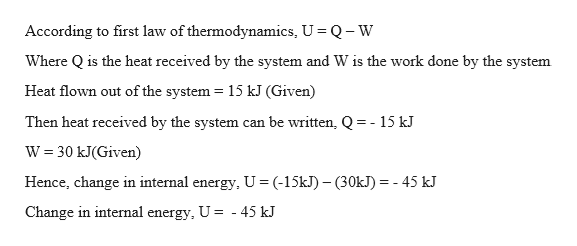# The first low of the thermodynamics shows U = Q - W, where U is a changing internal energy of the gas in the system, Q is a heat that the system received, and DW is work done by the system. If 15 kJ of heat flows out of a system and 30 kJ of work is done by the system, then what is the change in internal energy?

Question
4 views

The first low of the thermodynamics shows U = Q - W, where U is a changing internal energy of the gas in the system, Q is a heat that the system received, and DW is work done by the system. If 15 kJ of heat flows out of a system and 30 kJ of work is done by the system, then what is the change in internal energy?

check_circle

Step 1

Given:

Heal flown out of the system = 15 kJ

Work done by the system = 30 kJ

Step 2

Calculating the change in t...help_outlineImage TranscriptioncloseAccording to first law of thermodynamics, U Q-W Where Qis the heat received by the system and W is the work done by the system Heat flown out of the system 15 kJ (Given) Then heat received by the system can be written, Q= -15 kJ W 30 kJ(Given) Hence, change in internal energy U (-15k)30KJ -45 kJ Change in internal energy, U - 45 kJ fullscreen

### Want to see the full answer?

See Solution

#### Want to see this answer and more?

Solutions are written by subject experts who are available 24/7. Questions are typically answered within 1 hour.*

See Solution
*Response times may vary by subject and question.
Tagged in

### Thermodynamics